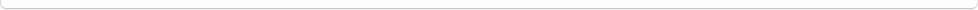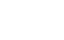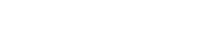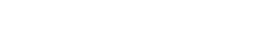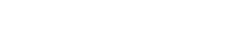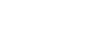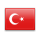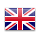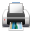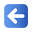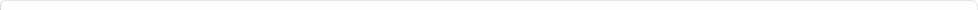Description of Individual Course Units
 Course Unit Code Course Unit Title Type of Course Unit Year of Study Semester Number of ECTS Credits 20150102001101 PHYSICS - I Compulsory 1 1 5
Level of Course Unit
First Cycle
Objectives of the Course
1. to be able to write equations of motion for simple mechanical systems, and to integrate these equations. 2. In cases where direct integration is not feasible, students are expected to make predictions based on conservation laws. 3. To apply Newton’s laws and conservation laws to dynamical systems.
Name of Lecturer(s)
Doç. Dr. Gökhan Şahin
Learning Outcomes
 1 1. Basic operation with vectors (additíon, scalar and vectorial product) 2 2. Kinematics in one and two dimesions 3 3. Application of Newton's laws to fundemental problems of mechanics 4 4. Concept of conservative force 5 5. Mechanics of rigid bodies rotating about a fixed axis and gyroscope motion 6 6. Using conservation laws when direct integration of motion is not feasible 7 7. Applications of conservation laws to dynamical systems.
Mode of Delivery
Daytime Class
Prerequisites and co-requisities
None
Recommended Optional Programme Components
None
Course Contents
Units, Physical Quantities, and Vectors. Motion Along a Straight Line. Motion in Two or Three Dimensions. Newton's Laws of Motion. Applying Newton's Laws. Work and Kinetic Energy. Potential Energy and Energy Conservation. Momentum, Impulse, and Collisions. Rotation of Rigid Bodies. Dynamics of Rotational Motion. Equilibrium and Elasticity.
Weekly Detailed Course Contents
 Week Theoretical Practice Laboratory 1 1 Units and Physical Quantities: Fundamental quantities, significant figures. 2 Vectors: Addition of vectors, scalar product, vector productI 3 Motion in one and two dimensions: Position, velocity and acceleration vectors, projectile motion, uniform circular motion. 4 Newton’s Laws: 1., 2., and 3. Newton’ s law. 5 Application of Newton laws: Friction, uniform circular motion. 6 Work and Kinetic Energy: Work, Work-energy theorem, power. 7 Potential energy and conservation of energy: Conservative and non-conservative forces. 8 Momentum and motion of system of particle: Center of mass, motion of the center of mass. 9 Momentum and motion of system of particle: Conservation of momentum. 10 Rotational kinematics: Angular position, angular velocity, angular acceleration, moment of inertia. 11 Rotational dynamics: Torque, angular momentum 12 Rotational dynamics: Rotation of rigid a body, work concept for rotation, conservation of angular momentum, gyroscopes. 13 Static Equilibrium of Rigid Bodies: Conditions for static equilibrium. 14 Elasticity: Stress, strain.
1. FİZİK, WE Gettys, FJ Keller, MJ Skove (çeviri), Mc Graw Hill & Literatür Yayıncılık. 2. PHYSICS, PM Fishbane, S Gasiorowicz, ST Thornton, 3rd ed., Pearson Pub. 3. TEMEL FİZİK Cilt 1, PM Fishbane, S Gasiorowicz, ST Thornton (çeviri), Arkadaş Yayıncılık. 4. FİZİK Cilt 1, R Serway (çeviri) Palme Yayıncılık. 5. FİZİK, DC Giancoli (çeviri), 4. Baskı, Pearson-Printice Hall & Arkadaş Yayıncılık 6. Fen ve Mühendislik için Fizik 1, Serway-Beichner.,
Planned Learning Activities and Teaching Methods
Assessment Methods and Criteria
 Term (or Year) Learning Activities Quantity Weight Midterm Examination 1 100 SUM 100 End Of Term (or Year) Learning Activities Quantity Weight Final Examination 1 100 SUM 100 Term (or Year) Learning Activities 50 End Of Term (or Year) Learning Activities 50 SUM 100
Language of Instruction
Turkish
Work Placement(s)
None